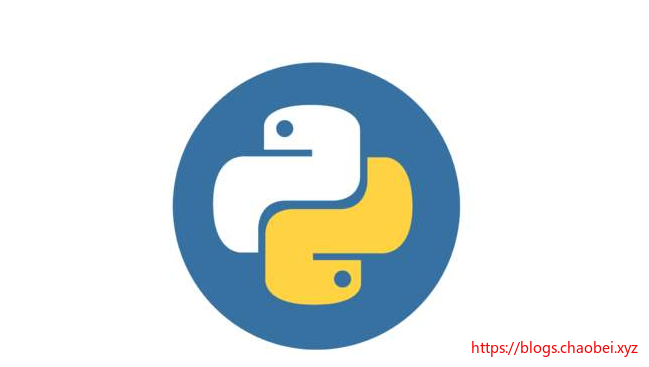# PYTHON 学习笔记4 模块的使用、基本IO 写入读取、JSON序列化

## 尝试

### 创建一个项目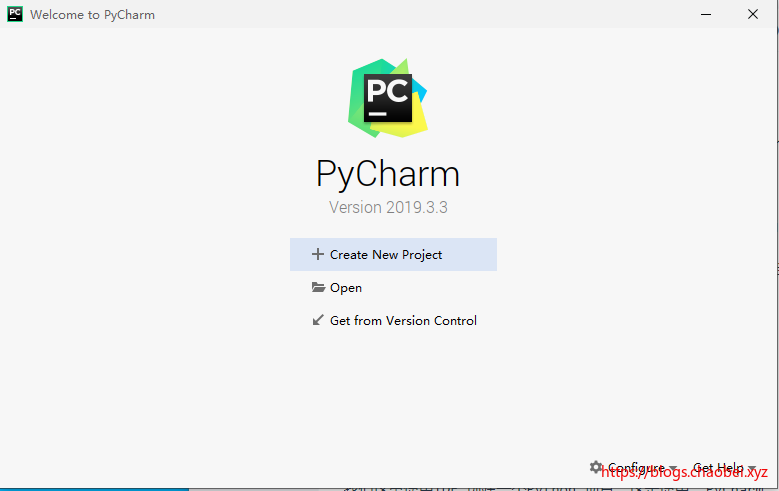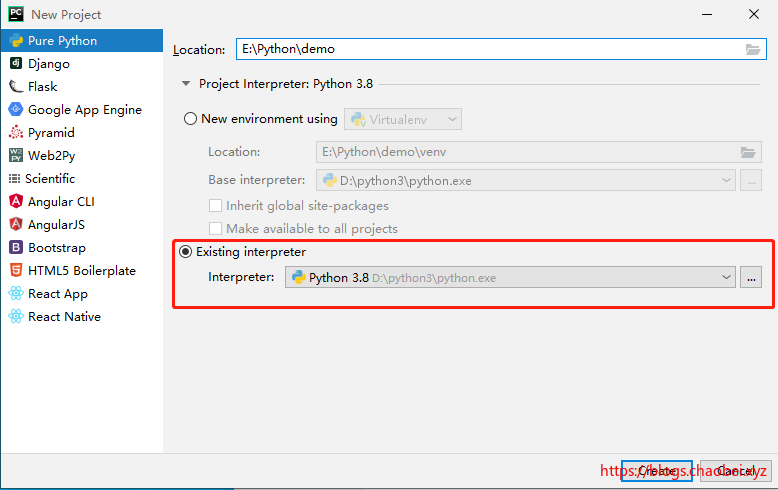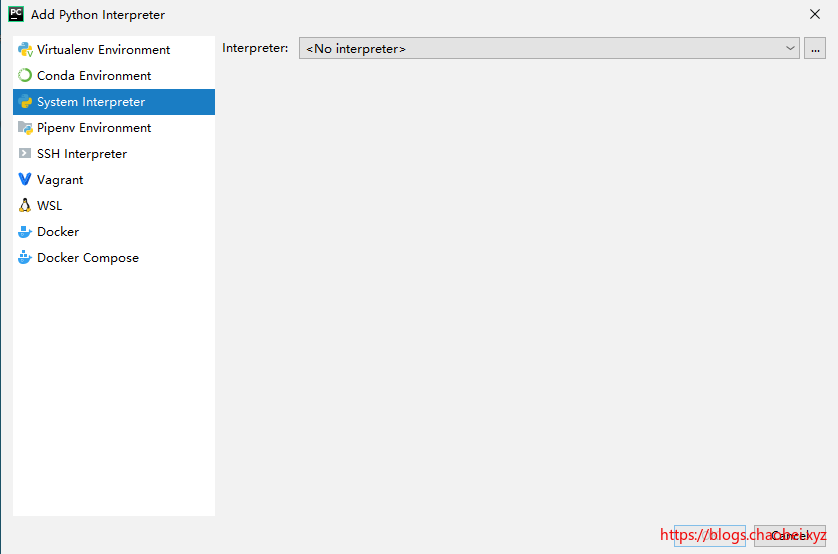### 创建一个模块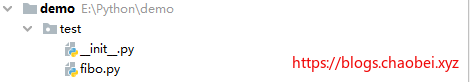``````def fib(n):    # write Fibonacci series up to n
a, b = 0, 1
while a < n:
print(a, end=' ')
a, b = b, a+b
print()

def fib2(n):   # return Fibonacci series up to n
result = []
a, b = 0, 1
while a < n:
result.append(a)
a, b = b, a+b
return result
``````

``````import fibo as fi

fi.fib(100)
----------------
0 1 1 2 3 5 8 13 21 34 55 89
``````

### 另一种导入

``````from fibo import fib,fib2

fib(100)
---------------
0 1 1 2 3 5 8 13 21 34 55 89
``````

### 模块搜索路径

``````import sys

print(sys.path)
-----------------
['E:\\Python\\demo','D:\\python3\\DLLs', 'D:\\python3\\lib', 'D:\\python3', 'D:\\python3\\lib\\site-packages']
``````

• 工作目录
• 以及Python 安装目录下的DLLS/lib/site-packages 这些目录

## 建立Python 包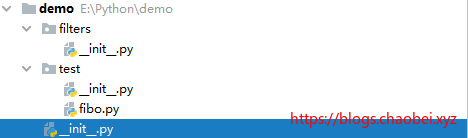``````import test.fibo as fib

print(dir(fib))
---------------
['__builtins__', '__cached__', '__doc__', '__file__', '__loader__', '__name__', '__package__', '__spec__', 'fib', 'fib2']
``````

## 输出输出

### 格式化字符串

``````year = 2020
event = 'Python'

test = f'hello world in {year} study {event}'

print(test)
------------------
hello world in 2020 study Python
``````

### 控制最小宽度

``````import math
print(f'The value of pi is approximately {math.pi:.3f}.')
-----------------
The value of pi is approximately 3.142.
``````

### format() 方法

``````str = 'hello {} my name is {}'

print(str.format('python','helloworld'))
-----------------------
hello python my name is helloworld
``````

## 读写文件

open() 返回一个 file object，最常用的有两个参数： `open(filename, mode)`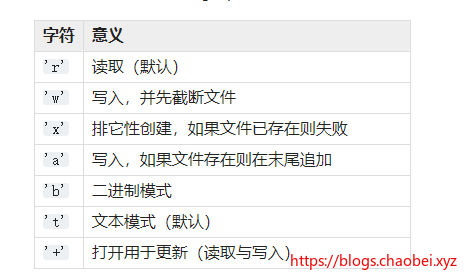``````with open('E:\\Python\\demo\\file.txt') as f:

----------
hello world
``````

``````with open('E:\\Python\\demo\\file.txt') as f:
for line in f:
print(line,end='')
------------------
hello world
Python
``````

### 写入文件

`f.write(string)` 会把 string 的内容写入到文件中，并返回写入的字符数。这里使用的模式是`r+` 其实就是同时可以进行文件的读取和写入。但是写入的内容需要在文件关闭后才可以读取出来，不能同时读取写入。

``````with open('E:\\Python\\demo\\file.txt','r+') as f:

strs = 'new line in write'

f.write(strs)
----------------

new line in write
``````

## JSON 数据

JSON 数据在后端也算是很优秀的。不管是字符/数组/数字/日期，他都可以使用字符串的形式存储起来。可以直接转换为对象供我们直接使用。用起来相当的方便啊。

Python 允许你使用称为 JSON (JavaScript Object Notation) 的流行数据交换格式，而不是让用户不断的编写和调试代码以将复杂的数据类型保存到文件中。

• 对象->json字符串： serialzing
• json字符串-> 对象： deserializing

### dumps（obj）

``````import json

obj = [1, 'simple', 'list']

print(type(obj))
str = json.dumps(obj)
print(str,'type',type(str))
--------------
<class 'list'>
[1, "simple", "list"] type <class 'str'>
``````

### dump(x,f)

• x 表示输入一个对象
• f 将对象序列保存到一个文本文件中

``````import json

obj = [1, 'simple', 'list']
with open('E:\\Python\\demo\\file.txt','r+') as f:

json.dump(obj,f)
----------------------

[1, "simple", "list"]
``````

``````import json

obj = [1, 'simple', 'list']
with open('E:\\Python\\demo\\file.txt','r+') as f:

print(obj,'type',type(obj))
----------
[1, 'simple', 'list'] type <class 'list'>

``````

## 参考

https://docs.python.org/zh-cn/3/